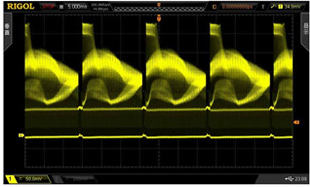Close
(0) Shopping cart
You have no items in your shopping cart.
Shopping Categories
Search

# Analog Bandwidth VS Digital Real-time Bandwidth

Bandwidth is one of the most important indexes of oscilloscope. The bandwidth of analog oscilloscope is a fixed value, while the bandwidth of digital oscilloscope has two kinds: analog bandwidth and digital real-time bandwidth. The maximum bandwidth that digital oscilloscope can achieve by using sequential sampling or random sampling technology for repeated signals is the digital real-time bandwidth of oscilloscope. The digital real-time bandwidth is related to the maximum digital frequency and waveform reconstruction technology factor K (digital real-time bandwidth = maximum digital rate / k), which is generally not directly given as an index.

Difference of the two kinds of bandwidth

From the definition of the two kinds of bandwidth, it can be seen that the analog bandwidth is only suitable for the measurement of repetitive signal, while the digital real-time bandwidth is suitable for the measurement of repetitive signal and single signal at the same time. Manufacturers claim that the bandwidth of the oscilloscope can reach how many megabytes, actually refers to the analog bandwidth, digital real-time bandwidth is lower than this value. For example, the bandwidth of tes520b is 500MHz, which actually means that its analog bandwidth is 500MHz, while the maximum digital real-time bandwidth can only reach 400MHz, far lower than the analog bandwidth .So in the measurement of single signal, we must refer to the digital real-time bandwidth of digital oscilloscope, and otherwise it will bring unexpected error to the measurement. About sampling rate. Sampling rate, also known as digital rate, refers to the sampling times of analog input signal per unit time, often expressed in MS / s. Sampling rate is an important index of digital oscilloscope.If the sampling rate is not enough, aliasing is easy to occur

If the input signal of the oscilloscope is a 100 KHz sinusoidal signal, but the signal frequency displayed by the oscilloscope is 50 KHz, what's the matter? This is because the sampling rate of oscilloscope is too slow, resulting in aliasing phenomenon. Aliasing means that the waveform frequency displayed on the screen is lower than the actual frequency of the signal, or even if the trigger indicator on the oscilloscope is on, the displayed waveform is still unstable .So, for a waveform with unknown frequency, how to judge whether the displayed waveform has generated aliasing? By slowly changing the scan speed T / div to a faster time base, we can see whether the frequency parameters of the waveform change sharply. If so, it indicates that waveform aliasing has occurred; or if the swaying waveform stabilizes at a faster time base, it also indicates that waveform aliasing has occurred. According to Nyquist theorem, only when the sampling rate is at least twice higher than the high frequency component of the signal can aliasing not occur. For example, a 500MHz signal needs at least 1gs / s sampling rate.

There are several methods to prevent aliasing

• Adjusting the scan speed
• Using AUTOSET Adjusting the scan speed, Try to switch the collection mode to envelope mode or peak detection mode, because envelope mode is to find the extreme value in multiple collection records, while peak detection mode is to find the maximum and minimum value in a single collection record. Both methods can detect faster signal changes.
• If the oscilloscope has instavu acquisition mode, it can be selected, because this mode has fast acquisition speed, and the waveform displayed by this method is similar to that displayed by analog oscilloscope.

The relationship between sampling rate and T / div

• The maximum sampling rate of each digital oscilloscope is a fixed value. However, at any scanning time t / div, the sampling rate FS is given by the following formula: FS = n / (T / div) n is the sampling point per grid. When the number of sampling points n is a certain value, FS is inversely proportional to t / Div. the larger the scanning speed is, the lower the sampling rate is.
• To sum up, when using digital oscilloscope, in order to avoid aliasing, it is better to put the sweep speed gear in the position with faster sweep speed. If you want to catch the fleeting burr, it's better to put the sweep speed gear in the position where the main sweep speed is slow.
Rise time of digital oscilloscope in analog oscilloscope, rise time is an extremely important indicator of oscilloscope. In the digital oscilloscope, the rise time is not even given as an indicator. Due to the measurement method of digital oscilloscope, the rise time measured automatically is not only related to the position of sampling point.
• Although the rise time of waveform is a fixed value, the results measured by digital oscilloscope are quite different because of different scanning speed. The rise time of analog oscilloscope has nothing to do with the sweep speed, while the rise time of digital oscilloscope is not only related to the sweep speed, but also related to the position of sampling point. When using digital oscilloscope, we can't deduce the rise time of signal according to the measured time just like using analog oscilloscope.